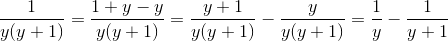## Example Questions

### Example Question #168 : Fractions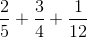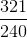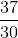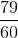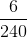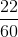Explanation:

To add fractions you first must find the lowest common denominator. For these fractions it is 60. Then you must multiply the numerator and denominator by the number such that the denominator is equal to the LCD. For examplegets multiplied by 12 (on both the numerator and denominator) because 5 times 12 is 60. When you do that you get the expression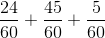then you just add the numerators and get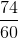2 goes into both of those numbers so you get### Example Question #171 : Fractions

Simplify the expression: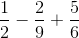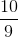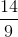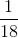Explanation:

First you want to find the least common denominator (in this case it's 18):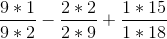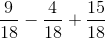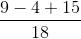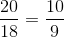### Example Question #1 : How To Add Fractions

What is the result of addingofto?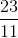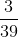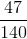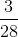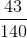Explanation:

Let us first get our value for the percentage of the first fraction. 20% of 2/7 is found by multiplying 2/7 by 2/10 (or, simplified, 1/5): (2/7) * (1/5) = (2/35)

Our addition is therefore (2/35) + (1/4). There are no common factors, so the least common denominator will be 35 * 4 or 140. Multiply the numerator and denominator of 2/35 by 4/4 and the numerator of 1/4 by 35/35.

This yields:

(8/140) + (35/140)  = 43/140, which cannot be reduced.

### Example Question #172 : Fractions

Which of the following is equal to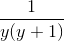?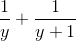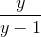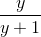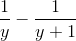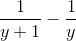We have two options here. We can manipulate the answers to work backwards and find a common denominator. This involves simply subtracting or adding two fractions. We can also try to rewrite the numerator by adding and subtracting the value. This serves the purpose of creating a sum in the numerator than can be split into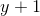and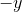. This gives us one of the factors in the denominator in each numerator. When we separate or decompose the fraction, we can divide out by the common factor to re-express this as the difference of two rational expressions.